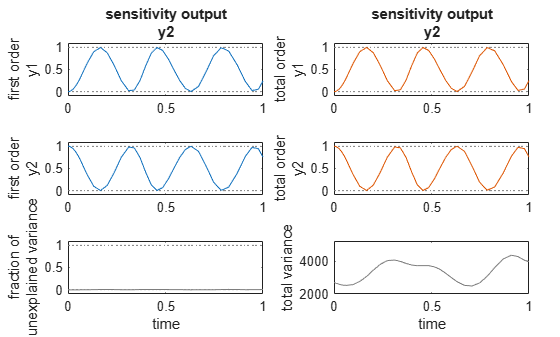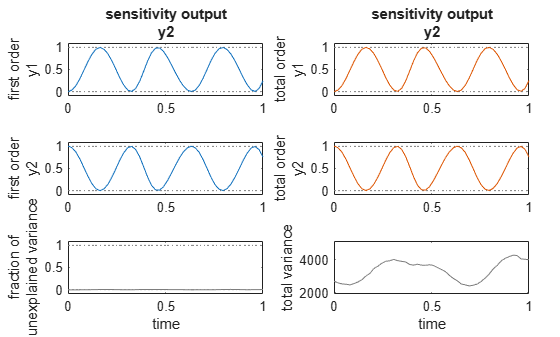# resample

Resample Sobol indices to new time vector

## Syntax

``results = resample(sobolObj,timeVector)``
``results = resample(sobolObj,timeVector,method)``

## Description

example

````results = resample(sobolObj,timeVector)` resamples model evaluations to a vector of new time points. By default, the function uses the `interp1q` interpolation method.```

example

````results = resample(sobolObj,timeVector,method)` specifies the interpolation `method`.```

## Examples

collapse all

`m = sbmlimport("lotka");`

Decompose the variance of predators y2 into attributions of the initial values of the prey y1 and predators.

```sobolResults = sbiosobol(m,["y1","y2"],"y2","StopTime",1); plot(sobolResults);```Resample the Sobol indices to a new time vector.

```newSobolResults = resample(sobolResults,linspace(0,1,50)); plot(newSobolResults);```## Input Arguments

collapse all

Results containing the first- and total-order Sobol indices, specified as a `SimBiology.gsa.Sobol` object.

New time points, specified as a nonempty real numeric vector containing finite and increasing values.

If `timeVector` includes time points outside the time interval encompassed by the simulation data in `sobolObj`, `resample` performs extrapolation. The function issues a warning and throws an error if resampling fails due to extrapolation.

See the help for the MATLAB function corresponding to the interpolation method in use for information on how the function performs the extrapolation.

Data Types: `double`

Interpolation method, specified as a string or character vector. The valid options follows.

• `'interp1q'` — Use the `interp1q` function.

• Use the `interp1` function by specifying one of the following methods:

• `'nearest'`

• `'linear'`

• `'spline'`

• `'pchip'`

• `'v5cubic'`

• `'zoh'` — Specify the zero-order hold.

Data Types: `char` | `string`

## Output Arguments

collapse all

Resampled simulation results with Sobol indices computed at new time points, returned as a `SimBiology.gsa.Sobol` object.

 Saltelli, Andrea, Paola Annoni, Ivano Azzini, Francesca Campolongo, Marco Ratto, and Stefano Tarantola. “Variance Based Sensitivity Analysis of Model Output. Design and Estimator for the Total Sensitivity Index.” Computer Physics Communications 181, no. 2 (February 2010): 259–70. https://doi.org/10.1016/j.cpc.2009.09.018.

## SupportGet trial now